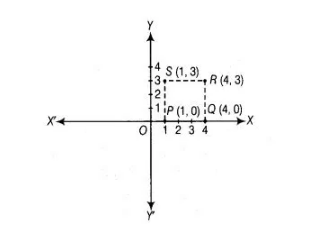# Plot the points P(1, 0), Q(4, 0) and 5(1, 3).`
Question:

Plot the points P(1, 0), Q(4, 0) and 5(1, 3). Find the coordinates of the point R such that PQRS is a square.

Solution:

In point P( 1, 0), y-coordinate is zero, so it lies on X-axis. In point Q(4, 0), y-coordinate is zero so it lies on X-axis. In point S (1, 3), both coordinates are positive, so it lies in I quadrant. On plotting these points, we get the following graph.Now, take a point R on the graph such that PQRS is a square. Then, all sides will be equal i.e., PQ = QR= RS = PS. So, abscissa of R should be equal to abscissa of Q i.e., 4 and ordinate of R should be equal to ordinate of S i.e., 3. Hence, the coordinates of R are (4, 3).